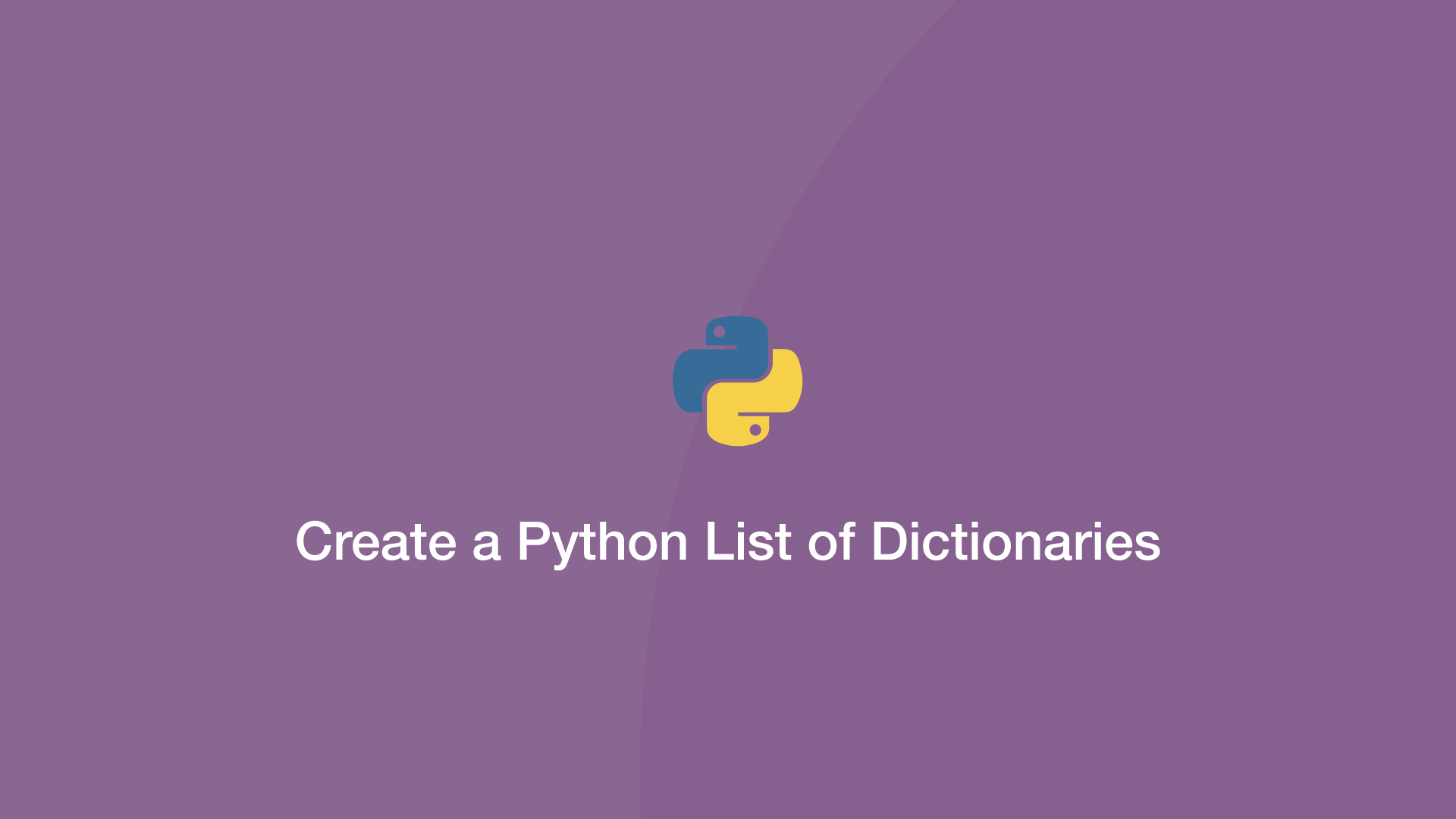# How to Create a Python List of DictionariesTo create a list of dictionaries in Python, pass them as a comma-separated list inside `[]` (square brackets) and store the result in a variable.

Let's try this out with an example.

``````dict1 = {'a':1, 'b':2}
dict2 = {'c':3, 'd':4}

items = [dict1, dict2]

print(items)
``````
``````[{'a': 1, 'b': 2}, {'c': 3, 'd': 4}]
``````

## Add Dictionary to Existing List

To add dictionaries as elements in an existing Python list, use the `.append()` function like this:

``````dict1 = {'a':1, 'b':2}
dict2 = {'c':3, 'd':4}

items = []

items.append(dict1)
items.append(dict2)

print(items)
``````
``````[{'a': 1, 'b': 2}, {'c': 3, 'd': 4}]
``````

## Accessing Dictionary Values within a List

To access the value of a dictionary list item, access the index of the dictionary first, then the dictionary key (both in separated square brackets.)

``````items = [{'a': 1, 'b': 2}, {'c': 3, 'd': 4}]

b = items['b']

print(b)
``````
``````2
``````

## Update Dictionary Values in a List

To update a dictionary value that's in a list, use the same access method as above, then set the value using the  `=` (equals) operator.

``````items = [{'a': 1, 'b': 2}, {'c': 3, 'd': 4}]

items['b'] = 10

b = items['b']

print(b)
``````
``````10
``````

#### Related Tutorials### How to Read CSV Files in Python

October 02, 2020### How to Use Python List Comprehension

October 04, 2020### How to Sort Dictionaries by Value In Python

September 22, 2020### How to use the Python filter() Function

September 28, 2020### How to Parse JSON Data in Python (Read and Write)

September 18, 2020April 13, 2021